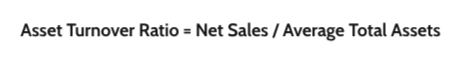Resources

Resources

Calculate Average Asset

Total Asset Turnover# Total asset turnover calculator

The asset turnover ratio calculator helps you easily calculate the asset turnover ratio. This metric measures how efficiently a company utilizes its assets to generate sales or revenue. A larger ratio suggests that the company is more effective in generating sales or revenue. While on the other end, a lower ratio means that the company may not be utilizing its assets effectively.

This article will define the vocabulary associated with the asset turnover ratio and will allow you to understand how to calculate the asset turnover ratio correctly. The article will finish by diving into a step-by-step example of the asset turnover ratio and how it looks in the application.

You can also look at our other financial calculators, for example, the debt-to-asset ratio (especially useful for companies) or the debt-to-income ratio (interesting for personal finance purposes).

## What is the asset turnover ratio?

The asset turnover ratio is used to measure how efficiently a company utilizes its assets to generate revenue. It’s a metric that is used to compare the performance of the company’s income statement with the company’s balance sheet.

What is a good asset turnover ratio?

An asset turnover ratio that displays a higher value means that a company is better at utilizing assets to generate revenue. On the other hand, a lower asset turnover ratio suggests that a company is failing to use its assets effectively. The last section of this article will display two examples of companies with two very different asset turnover ratios.

## Asset turnover ratio formula

The asset turnover ratio measures the performance of a company’s income statement with the company’s financial security on the balance sheet. In formula form, the asset turnover ratio looks like this:Net sales are a company’s gross sales minus allowances, discounts, and returns. In other words, net sales is the total amount of revenue made by a company. The average total assets … The next section will dive into an example to show a clearer picture of how the asset turnover ratio works.

## How to calculate the asset turnover ratio

For this example, Company A has a total net sales of \$6.5 million at the end of its fiscal year. The company’s total assets at the beginning of the year were \$3 million and \$1.5 million at the end of the fiscal year. The average of the total assets are \$3.75 million ([\$3 million + \$1.5 million] / 2). Here’s how the formula looks when you enter the above values into the asset turnover ratio calculator:

Company A’s Asset Turnover Ratio = \$6.5 million / \$3.75 million = 1.73

The next company in the example is Company B with total revenue of \$7 million at the end of the fiscal year. Company B’s average total assets at the end of the year were \$1.5 million. Here’s how the values will appear in the formula:

Company B’s Asset Turnover Ratio = \$7 million / \$1.5 million = 4.67

These ratios suggest that Company B is more efficiently using its assets to generate revenue as its asset turnover ratio is greater than Company A’s asset turnover ratio. As mentioned previously, the higher the asset turnover ratio the better a company is utilizing its assets to generate revenue.## Matt here!

Your friends at EBizCharge have been building some great software that decreases days outstanding and lowers payment processing costs. You should check it out here.